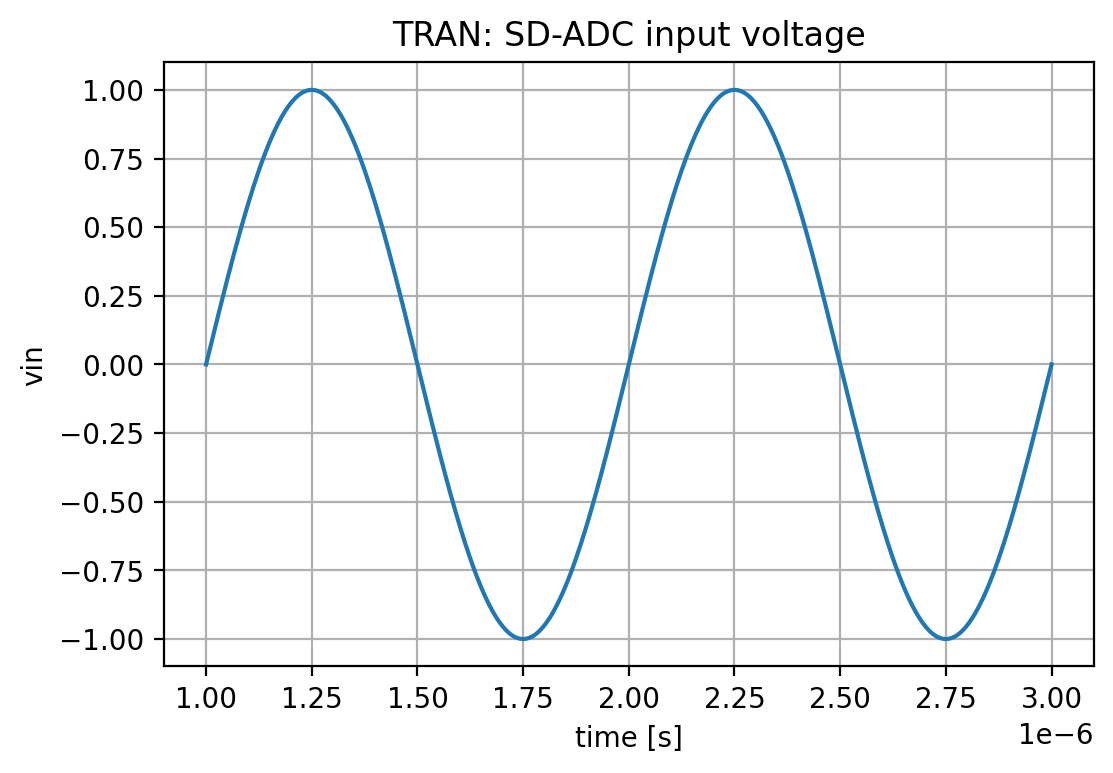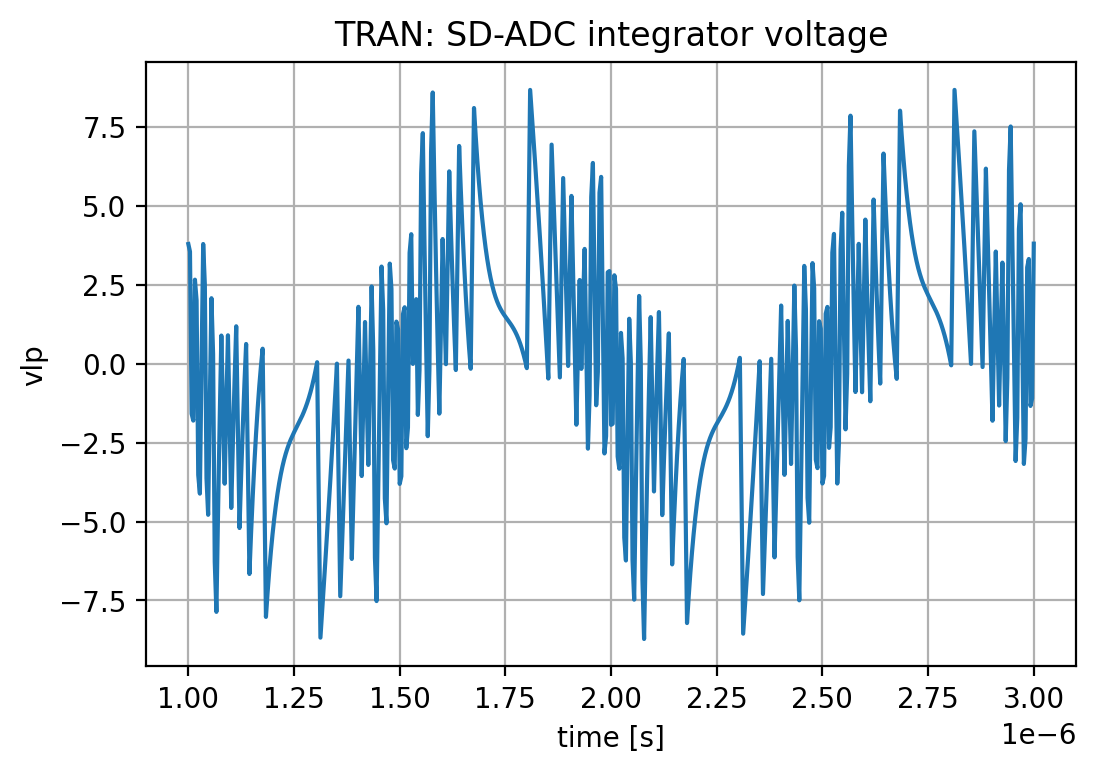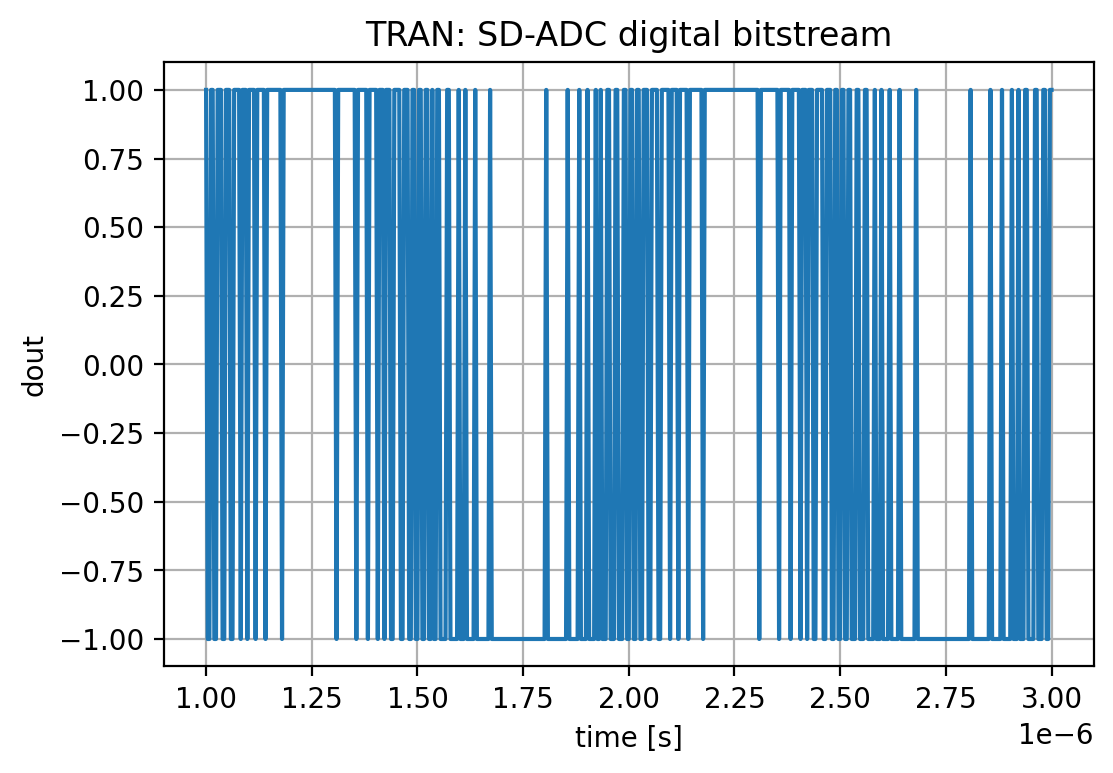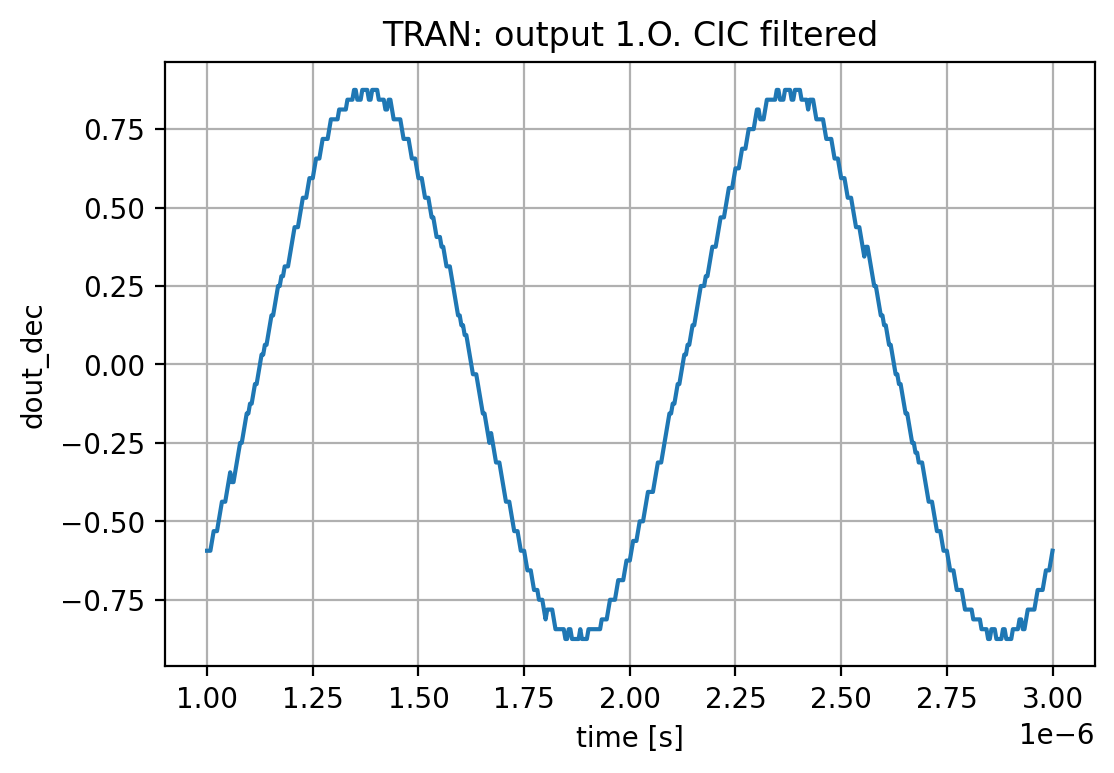# Continuous-Time Sigma Delta Converters

Author: Wolfgang Scherr
Date: 14th February 2021

(c) Carinthia University of Applied Sciences

SystemC-AMS models created with Coside.

# Data Converter Basics

This document does not elaborate on basics of signal processing and sampling or history of A/D converters. If you are interested in that, there is plenty of information on the web, e.g from Analog Devices here.

# Ideal continuous-time sigma-delta converter

For an ideal c.t. sigma-delta converter, an integrator is used. An equivalent SystemC-AMS (IEEE 1666.1) model using LSF (linear signal flow) looks like this:This leads to this signal transfer characteristics:

$$Hs(s) = \frac{Y(s)}{X(s)} = \frac{\frac{1}{s}}{1 + \frac{1}{s}} = \frac{1}{s + 1}$$

And to this noise transfer characteristics:

$$Hn(s) = \frac{Y(s)}{N(s)} = \frac{1}{1 + \frac{1}{s}}$$

Which can be nicely checked by frequency-domain simulations of the shown model:

Running : lsf_simple_simple_tb.exe --x_ac=1.0 --n_ac=0.0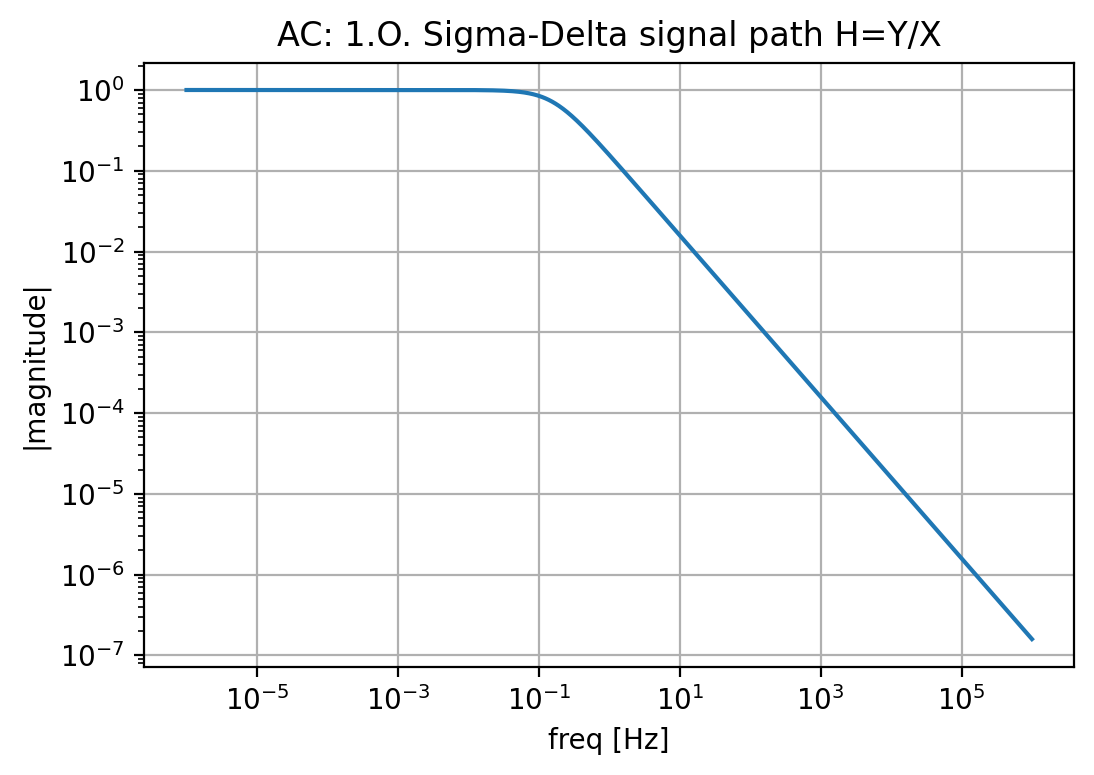Running : lsf_simple_simple_tb.exe --x_ac=0.0 --n_ac=1.0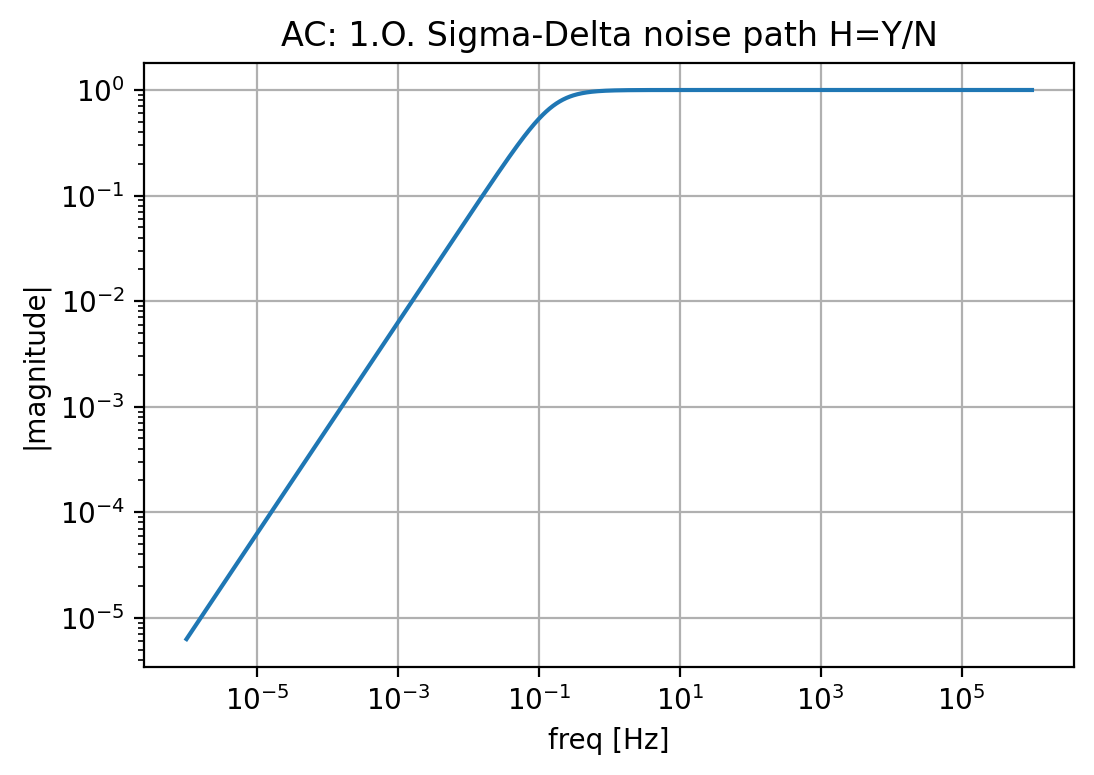This circuit has some problems. First of all, there is no ideal integrator in the analog world. In principle, they any implementation is more an active low-pass filter with some low corner frequency.

Secondly, the ideal integrator does not really provide a proper bandwidth to be used. For example: if we want a SD-ADC with 256MHz sampling rate to convert a 1MHz signal, we need more bandwidth for the signal path.

# A bit more realistic sigma-delta converter

Now we use a low-pass filter instead of an ideal integrator.The first order lowpass filter function is: $$Hlp(s) = \frac{1}{1 + \tau s}$$.

We set the corner frequency $$f_c$$ to about 2MHz ($$\tau = \frac{1}{2\pi f_c}$$). The transfer function of this low-pass filter alone will look as follows:

Tau = 7.957747154594767e-08
Hlp(s) = 1/(7.95774715459477e-8*s + 1)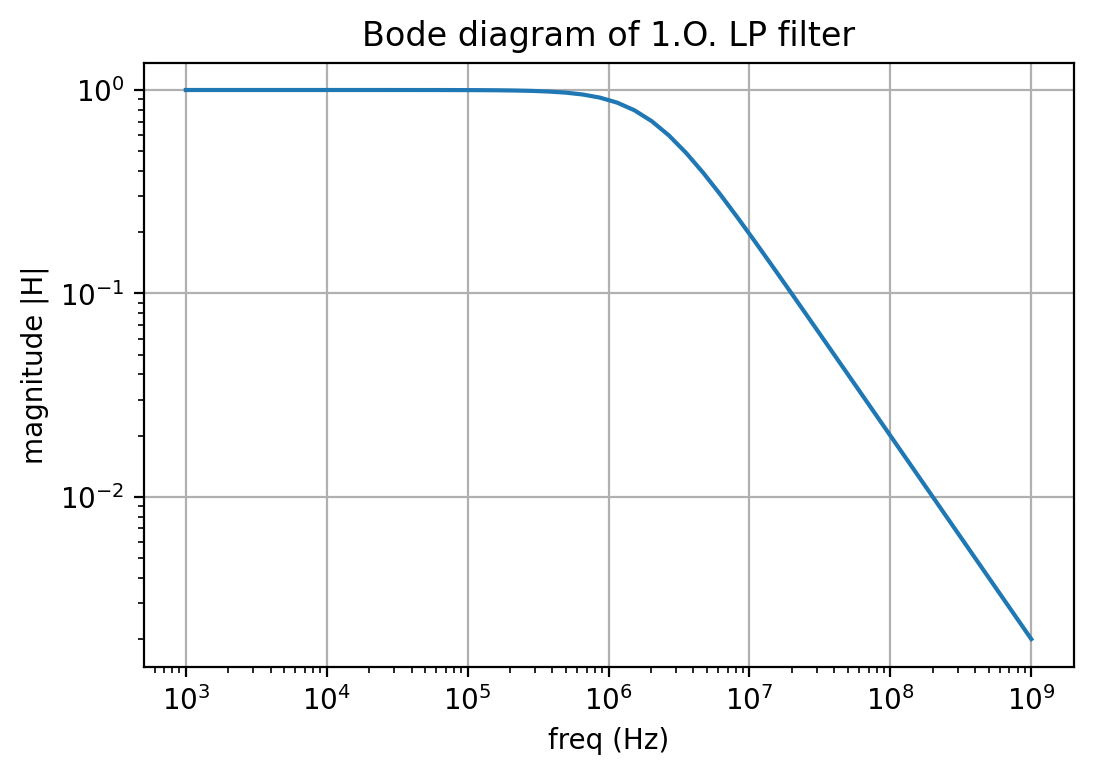In the closed loop setup of the SD-ADC we will now get for the signal transfer function:

$$Hs(s) = \frac{Y(s)}{X(s)} = \frac{\frac{1}{1+\tau s}}{1 + \frac{1}{1+\tau s}} = \frac{1}{\tau s + 2}$$

Looking at the noise transfer function we have now:

$$Hn(s) = \frac{Y(s)}{N(s)} = \frac{1}{1 + \frac{1}{1+\tau s}}$$

Which we again check by a frequency domain simulation:

Running : lsf_realistic_simple_tb.exe --x_ac=1.0 --n_ac=0.0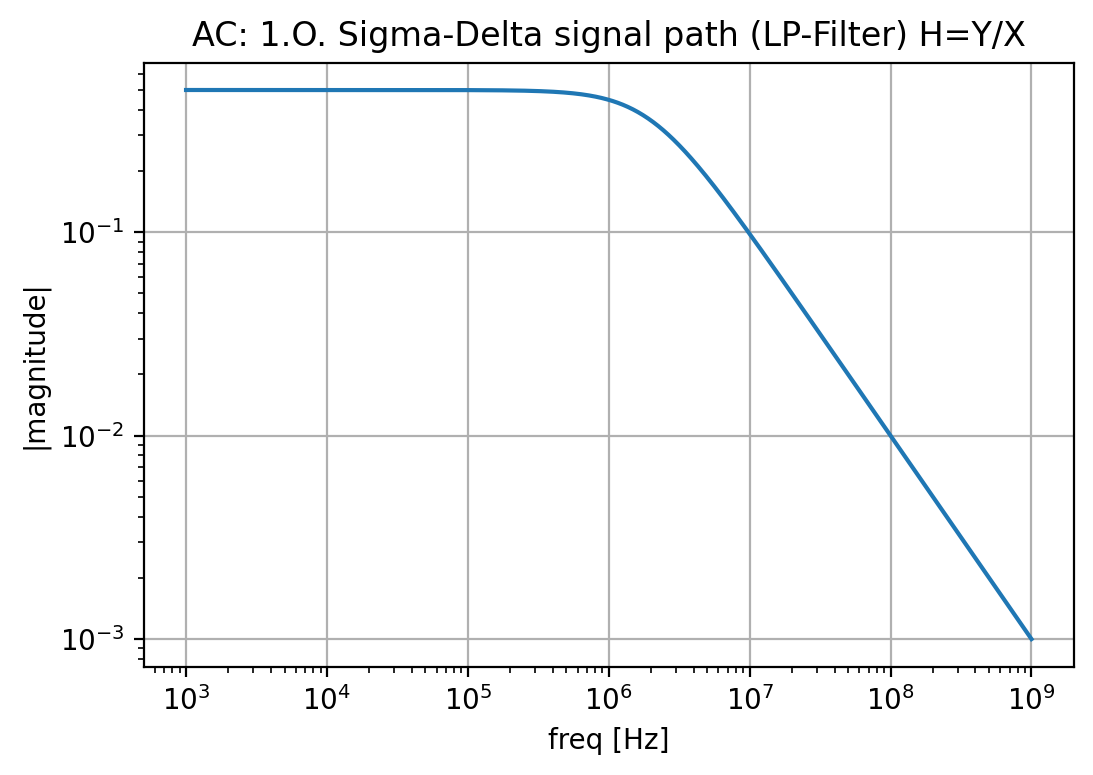Running : lsf_realistic_simple_tb.exe --x_ac=0.0 --n_ac=1.0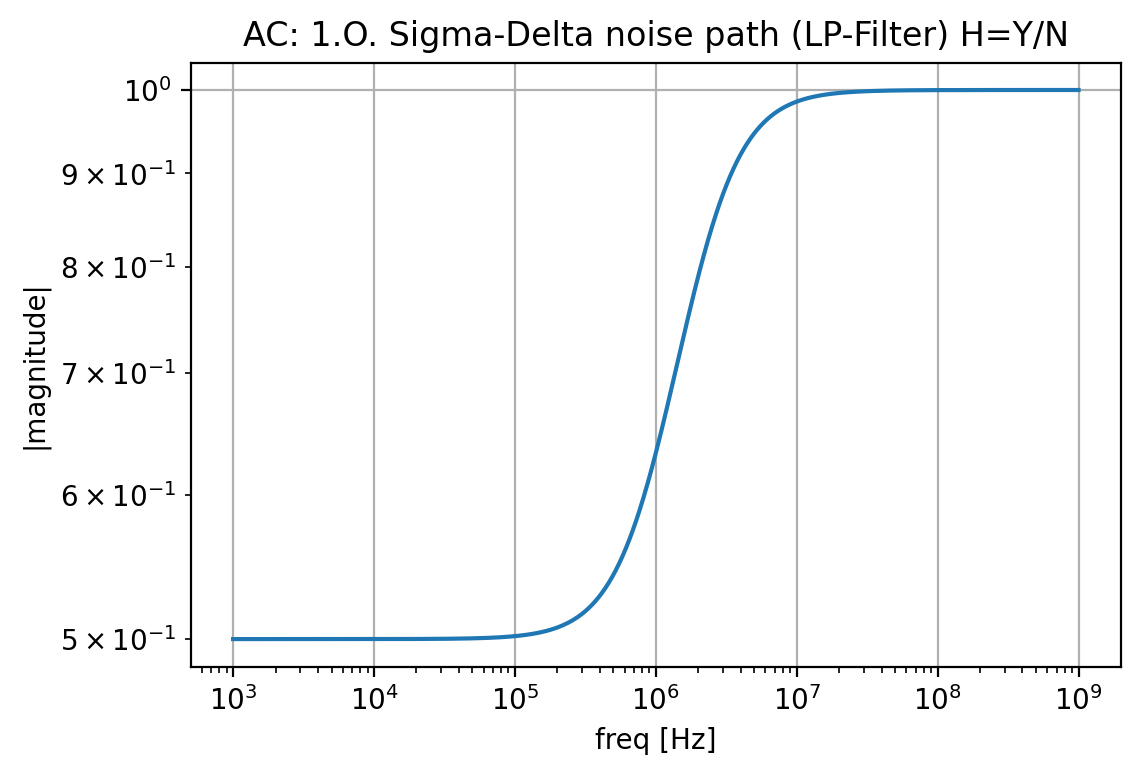This does not really like good noise shaping. So we need to do a bit more, which is introducing an active LP filter with some gain, looking at the filter alone we get now:

Tau = 7.957747154594767e-08
Hlp(s) = 100/(7.95774715459477e-8*s + 1)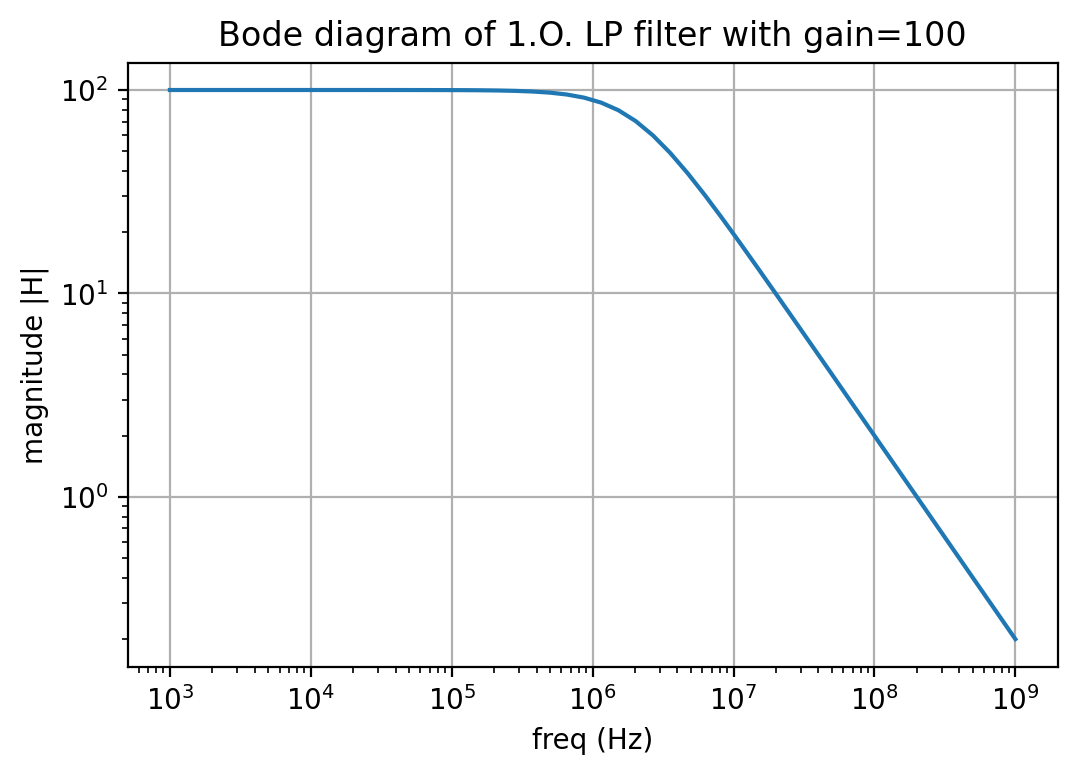What is now the effect in the closed-loop simulation of the converter? Let's check the frequency-domain simulation again:

Running : lsf_realistic_simple_tb.exe --x_ac=1.0 --n_ac=0.0
--gain=100.0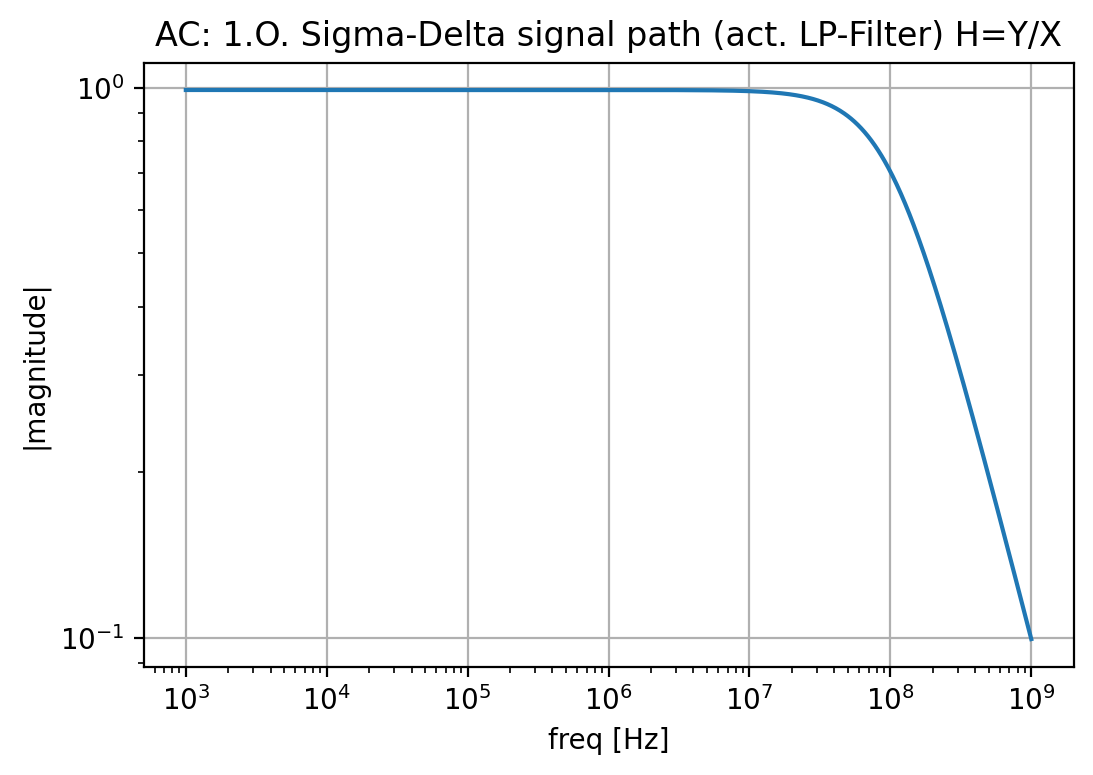Running : lsf_realistic_simple_tb.exe --x_ac=0.0 --n_ac=1.0
--gain=100.0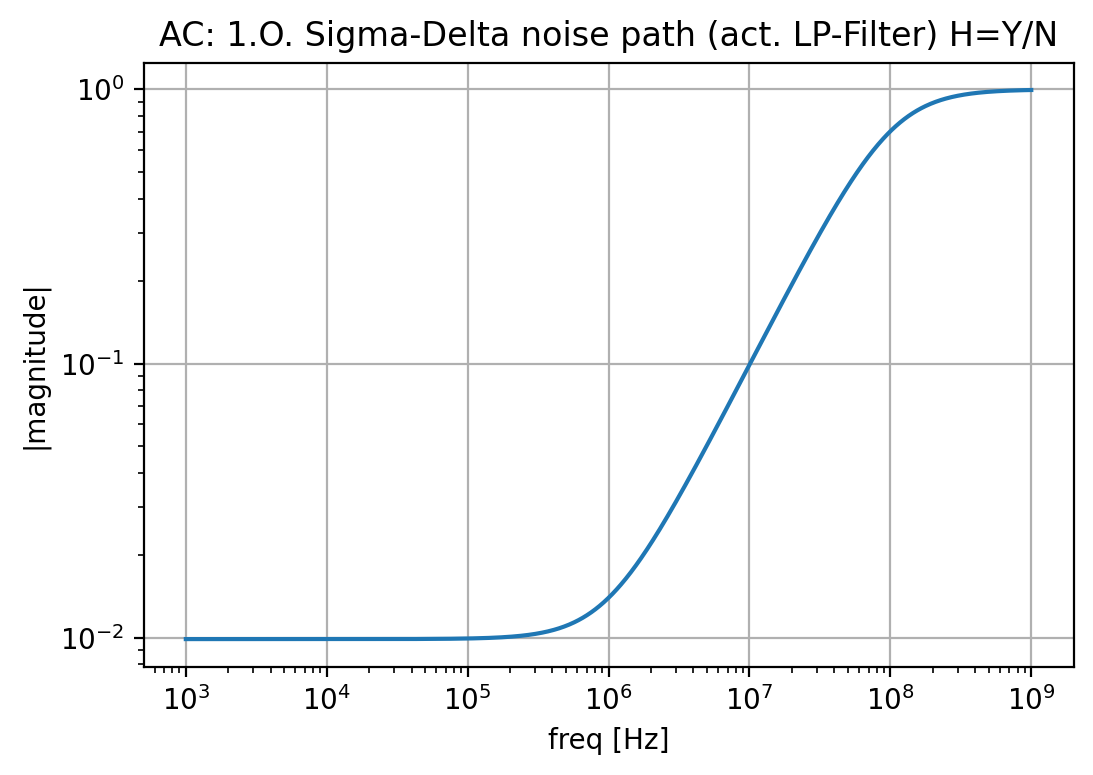Not so bad, right? We get a better signal bandwidth and shift the noise upwards, so really nice noise-shaping, even for a very simple 1.O. ct. SD-ADC...

# Ideal implementation model

But how does this look more on electrical level? Let's do a basic circuit model for our SD-ADC using SystemC-AMS (IEEE 1666.1) ELN (electrical linear network).

For that we define a simple 1.O. operational amplifier. The advantage of using SystemC-AMS for the model is, that it runs way faster than using SPICE, despite I can still use a SPICE-like entry many are familar with. Basically, it is nearly as fast as the LSF model we used before (we just have a bit larger network to solve)...Its frequency response simulated looks like this: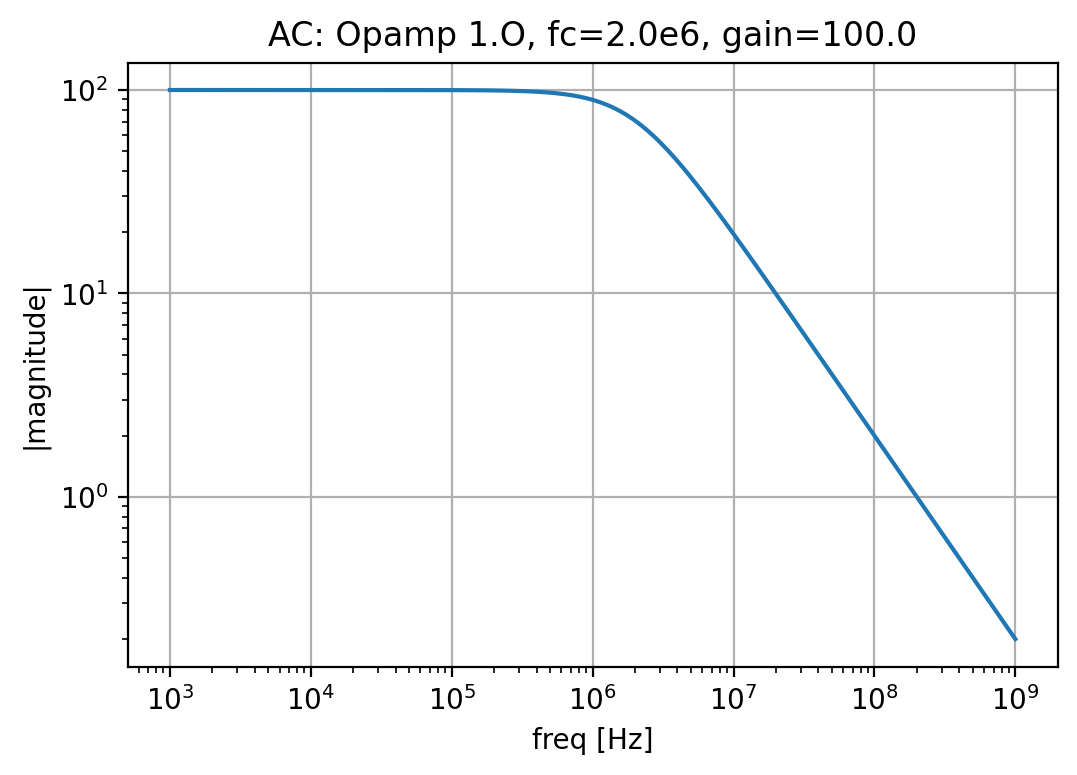The operational amplifier is included in the toplevel SD-ADC model. The output of the SD-ADC is connected to two 1.O. CIC with a frequency response corresponding to R=64, but we don't do the actual decimation to show the residual bins which would be folded back into the signal band.

For decimation, I used a simple averaging filter. More about decimation can be found on one of my other modelling pages here.Let's look at the result of a transient simulation of this model. For the FFT, I simulated 5ms, which took just a few seconds on my notebook. Here I plot just a few periods of the input signal. But be aware, the sample rate of the ADC is 256MHZ, so a lots of points to calculate and post-process.